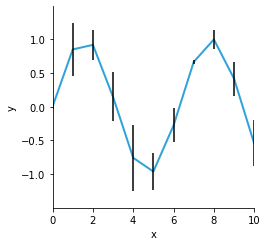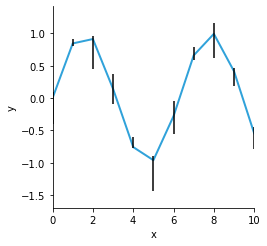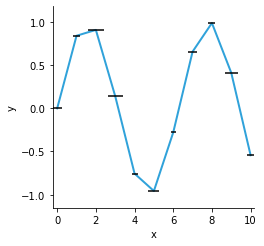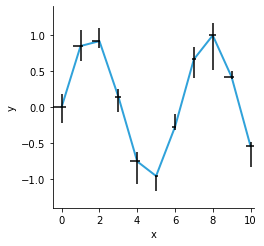# ErrorBars#

Title
ErrorBars Element
Dependencies
Matplotlib
Backends
Matplotlib
Bokeh
Plotly
```import numpy as np
import holoviews as hv
hv.extension('matplotlib')
````ErrorBars` provide a visual indicator for the variability of the plotted data on a graph. They are usually applied on top of other plots such as scatter, curve or bar plots to indicate the variability in each sample.

`ErrorBars` may be used to represent symmetric error or asymmetric error. An `ErrorBars` Element must have one key dimensions representing the samples along the x-axis and two or three value dimensions representing the value of the sample and positive and negative error values associated with that sample or x-axis. See the Tabular Datasets user guide for supported data formats, which include arrays, pandas dataframes and dictionaries of arrays.

## Symmetric error#

By default the `ErrorBars` Element accepts x- and y-coordinates along with a symmetric error value along y-axis:

```np.random.seed(7)
errors = [(0.1*i, np.sin(0.1*i), np.random.rand()/2) for i in np.linspace(0, 100, 11)]
hv.Curve(errors) * hv.ErrorBars(errors)
```## Asymmetric error#

`ErrorBars` is a set of x-/y-coordinates with associated error values along y-axis. Error values may be either symmetric or asymmetric, and thus can be supplied as an Nx3 or Nx4 array (or any of the alternative constructors Chart Elements allow).

```errors = [(0.1*i, np.sin(0.1*i), np.random.rand()/2, np.random.rand()/4) for i in np.linspace(0, 100, 11)]
hv.Curve(errors) * hv.ErrorBars(errors, vdims=['y', 'yerrneg', 'yerrpos'])
```## Errors along x-axis#

`ErrorBars` can be a set of a set of x-/y-coordinates with associated error values along x-axis. The parameter `horizontal`, when set to `True`, will set supplied errors along x-axis instead of y-axis.

```errors = [(0.1*i, np.sin(0.1*i), np.random.rand()/2) for i in np.linspace(0, 100, 11)]
hv.Curve(errors) * hv.ErrorBars(errors, horizontal=True)
```## Errors along x and y axes#

Two `ErrorBars` with orthogonal errors can be composed together to give x and y errorbars

```yerrors = [(0.1*i, np.sin(0.1*i), np.random.rand()/2, np.random.rand()/4) for i in np.linspace(0, 100, 11)]
xerrors = [(0.1*i, np.sin(0.1*i), np.random.rand()/2, np.random.rand()/4) for i in np.linspace(0, 100, 11)]

(hv.Curve(yerrors)
* hv.ErrorBars(yerrors, vdims=['y', 'yerrneg', 'yerrpos'])
* hv.ErrorBars(xerrors, vdims=['y', 'xerrneg', 'xerrpos'], horizontal=True)
)
```For full documentation and the available style and plot options, use `hv.help(hv.ErrorBars).`

This web page was generated from a Jupyter notebook and not all interactivity will work on this website. Right click to download and run locally for full Python-backed interactivity.